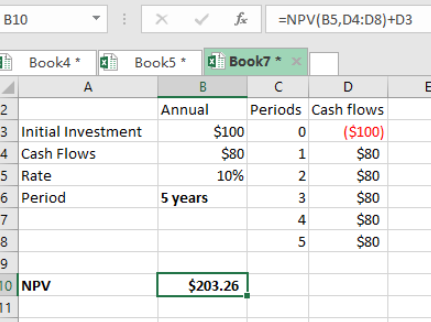Get instant live expert help with Excel or Google Sheets“My Excelchat expert helped me in less than 20 minutes, saving me what would have been 5 hours of work!”

#### Post your problem and you’ll get expert help in seconds.

Your message must be at least 40 characters
Our professional experts are available now. Your privacy is guaranteed.

# Here is How You Can Determine the NPV of Cash Flows in Excel

With the help of NPV function in excel, we can now determine the net present value of a given cash flow. This article provides a clear guide on how to use the NPV function when calculating the net present value.Figure 1. Final result

## General syntax of the formula

`=NPV(rate, cash flows)`

## Understanding the formula

We need to understand how the above formula works for us to use it when to determine the net present value of a given cash flow. Note that the cash flow given is constant, with a constant interval.

We also need to know the cost of capital, which is the interest rate in this case.

## How the formula works

The NPV function is fundamental when we want to find the NPV. The function relies heavily on the rate on interest that is supplied, plus the cash flows. It is important to note that in our example above, the rate is annual, and also the cash flows are annual.

We have used the following formula to get the NPV for the above cash flows;

`=NPV (B5,D4:D8)+D3`

Here, cell B5 refers to the rate of interest. The range D4:D8 refers to the periods, which is 5 years. Cell D3 refers to the initial investment, which is subtracted given that it is a cash outflow into the investment.

Most of the time, the problem you will need to solve will be more complex than a simple application of a formula or function. If you want to save hours of research and frustration, try our live Excelchat service! Our Excel Experts are available 24/7 to answer any Excel question you may have. We guarantee a connection within 30 seconds and a customized solution within 20 minutes.

Solution examplesI have a figure which is the sum of 9 months of payments. Each payment decreases by the same amount (X) so the first payment is Y, second payment is Y-X, third is Y-(Xx2), forth is Y-(Xx3) and so on. I am trying to calculate what each of the 9 payments should be.
Solved by A. W. in 60 mins